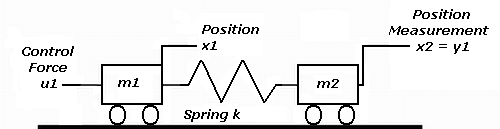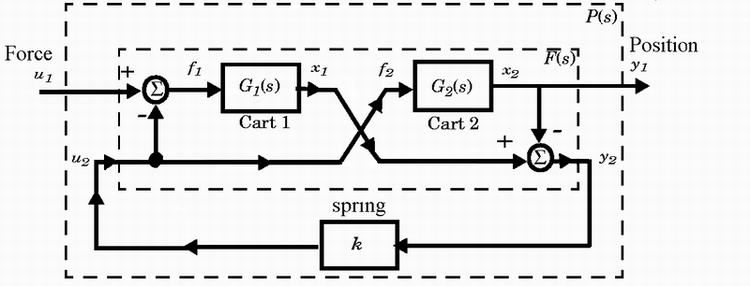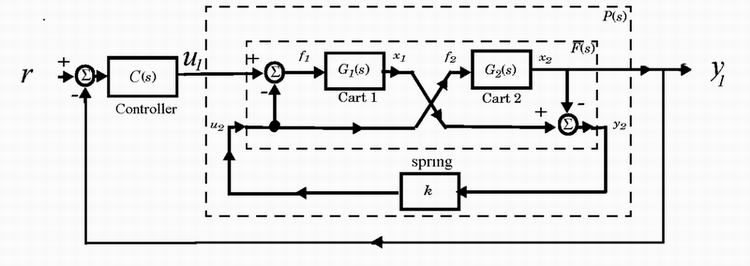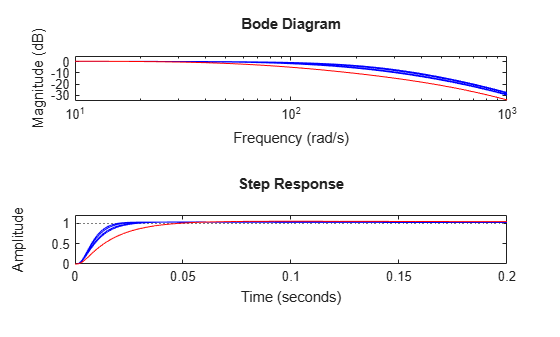# Building and Manipulating Uncertain Models

This example shows how to use Robust Control Toolbox™ to build uncertain state-space models and analyze the robustness of feedback control systems with uncertain elements.

We will show how to specify uncertain physical parameters and create uncertain state-space models from these parameters. You will see how to evaluate the effects of random and worst-case parameter variations using the functions `usample` and `robstab`.

### Two-Cart and Spring System

In this example, we use the following system consisting of two frictionless carts connected by a spring `k`:Figure 1: Two-cart and spring system.

The control input is the force `u1` applied to the left cart. The output to be controlled is the position `y1` of the right cart. The feedback control is of the following form:

`${u}_{1}=C\left(s\right)\left(r-{y}_{1}\right)$`

`$C\left(s\right)=100\left(s+1{\right)}^{3}/\left(0.001s+1{\right)}^{3}$`

We create this compensator using this code:

```s = zpk('s'); % The Laplace 's' variable C = 100*ss((s+1)/(.001*s+1))^3;```

### Block Diagram Model

The two-cart and spring system is modeled by the block diagram shown below.Figure 2: Block diagram of two-cart and spring model.

### Uncertain Real Parameters

The problem of controlling the carts is complicated by the fact that the values of the spring constant `k` and cart masses `m1,m2` are known with only 20% accuracy: $k=1.0±20%$ , $m1=1.0±20%$ , and $m2=1.0±20%$. To capture this variability, we will create three uncertain real parameters using the `ureal` function:

```k = ureal('k',1,'percent',20); m1 = ureal('m1',1,'percent',20); m2 = ureal('m2',1,'percent',20);```

### Uncertain Cart Models

We can represent the carts models as follows:

`${G}_{1}\left(s\right)=\frac{1}{{m}_{1}{s}^{2}},\phantom{\rule{0.2777777777777778em}{0ex}}\phantom{\rule{0.2777777777777778em}{0ex}}\phantom{\rule{0.2777777777777778em}{0ex}}{G}_{2}\left(s\right)=\frac{1}{{m}_{2}{s}^{2}}$`

Given the uncertain parameters `m1` and `m2`, we will construct uncertain state-space models (USS) for G1 and G2 as follows:

```G1 = 1/s^2/m1; G2 = 1/s^2/m2;```

### Uncertain Model of a Closed-Loop System

First we'll construct a plant model `P` corresponding to the block diagram shown above (`P` maps u1 to y1):

```% Spring-less inner block F(s) F = [0;G1]*[1 -1]+[1;-1]*[0,G2]```
```F = Uncertain continuous-time state-space model with 2 outputs, 2 inputs, 4 states. The model uncertainty consists of the following blocks: m1: Uncertain real, nominal = 1, variability = [-20,20]%, 1 occurrences m2: Uncertain real, nominal = 1, variability = [-20,20]%, 1 occurrences Type "F.NominalValue" to see the nominal value, "get(F)" to see all properties, and "F.Uncertainty" to interact with the uncertain elements. ```

Connect with the spring k

`P = lft(F,k)`
```P = Uncertain continuous-time state-space model with 1 outputs, 1 inputs, 4 states. The model uncertainty consists of the following blocks: k: Uncertain real, nominal = 1, variability = [-20,20]%, 1 occurrences m1: Uncertain real, nominal = 1, variability = [-20,20]%, 1 occurrences m2: Uncertain real, nominal = 1, variability = [-20,20]%, 1 occurrences Type "P.NominalValue" to see the nominal value, "get(P)" to see all properties, and "P.Uncertainty" to interact with the uncertain elements. ```

The feedback control u1 = C*(r-y1) operates on the plant `P` as shown below:Figure 3: Uncertain model of a closed-loop system.

We'll use the `feedback` function to compute the closed-loop transfer from r to y1.

```% Uncertain open-loop model is L = P*C```
```L = Uncertain continuous-time state-space model with 1 outputs, 1 inputs, 7 states. The model uncertainty consists of the following blocks: k: Uncertain real, nominal = 1, variability = [-20,20]%, 1 occurrences m1: Uncertain real, nominal = 1, variability = [-20,20]%, 1 occurrences m2: Uncertain real, nominal = 1, variability = [-20,20]%, 1 occurrences Type "L.NominalValue" to see the nominal value, "get(L)" to see all properties, and "L.Uncertainty" to interact with the uncertain elements. ```

Uncertain closed-loop transfer from r to y1 is

`T = feedback(L,1)`
```T = Uncertain continuous-time state-space model with 1 outputs, 1 inputs, 7 states. The model uncertainty consists of the following blocks: k: Uncertain real, nominal = 1, variability = [-20,20]%, 1 occurrences m1: Uncertain real, nominal = 1, variability = [-20,20]%, 1 occurrences m2: Uncertain real, nominal = 1, variability = [-20,20]%, 1 occurrences Type "T.NominalValue" to see the nominal value, "get(T)" to see all properties, and "T.Uncertainty" to interact with the uncertain elements. ```

Note that since `G1` and `G2` are uncertain, both `P` and `T` are uncertain state-space models.

### Extracting the Nominal Plant

The nominal transfer function of the plant is

`Pnom = zpk(P.nominal)`
```Pnom = 1 ------------- s^2 (s^2 + 2) Continuous-time zero/pole/gain model. ```

### Nominal Closed-Loop Stability

Next, we evaluate the nominal closed-loop transfer function `Tnom`, and then check that all the poles of the nominal system have negative real parts:

```Tnom = zpk(T.nominal); maxrealpole = max(real(pole(Tnom)))```
```maxrealpole = -0.8232 ```

### Robust Stability Margin

Will the feedback loop remain stable for all possible values of `k,m1,m2` in the specified uncertainty range? We can use the `robstab` function to answer this question rigorously.

```% Show report and compute sensitivity opt = robOptions('Display','on','Sensitivity','on'); [StabilityMargin,wcu] = robstab(T,opt);```
```Computing peak... Percent completed: 100/100 System is robustly stable for the modeled uncertainty. -- It can tolerate up to 288% of the modeled uncertainty. -- There is a destabilizing perturbation amounting to 289% of the modeled uncertainty. -- This perturbation causes an instability at the frequency 575 rad/seconds. -- Sensitivity with respect to each uncertain element is: 12% for k. Increasing k by 25% decreases the margin by 3%. 47% for m1. Increasing m1 by 25% decreases the margin by 11.8%. 47% for m2. Increasing m2 by 25% decreases the margin by 11.8%. ```

The report indicates that the closed loop can tolerate up to three times as much variability in `k,m1,m2` before going unstable. It also provides useful information about the sensitivity of stability to each parameter. The variable `wcu` contains the smallest destabilizing parameter variations (relative to the nominal values).

`wcu`
```wcu = struct with fields: k: 1.5773 m1: 0.4227 m2: 0.4227 ```

### Worst-Case Performance Analysis

Note that the peak gain across frequency of the closed-loop transfer `T` is indicative of the level of overshoot in the closed-loop step response. The closer this gain is to 1, the smaller the overshoot. We use `wcgain` to compute the worst-case gain `PeakGain` of `T` over the specified uncertainty range.

```[PeakGain,wcu] = wcgain(T); PeakGain```
```PeakGain = struct with fields: LowerBound: 1.0475 UpperBound: 1.0732 CriticalFrequency: 6.8070 ```

Substitute the worst-case parameter variation `wcu` into `T` to compute the worst-case closed-loop transfer `Twc`.

`Twc = usubs(T,wcu); % Worst-case closed-loop transfer T`

Finally, pick from random samples of the uncertain parameters and compare the corresponding closed-loop transfers with the worst-case transfer `Twc`.

```Trand = usample(T,4); % 4 random samples of uncertain model T clf subplot(211), bodemag(Trand,'b',Twc,'r',{10 1000}); % plot Bode response subplot(212), step(Trand,'b',Twc,'r',0.2); % plot step response```Figure 4: Bode diagram and step response.

In this analysis, we see that the compensator C performs robustly for the specified uncertainty on k,m1,m2.